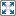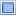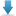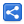# TypeScript - Using keyof to define permitted property names

[Last Updated: Oct 26, 2018]

The operator keyof helps us to use correct property name where it is needed.

keyof is an indexed type query which yields the type of permitted property names for an object.

The keyof type is considered a subtype of string.

# Examples

```class A {
x: number = 5;
}

let y: keyof A;
y = "x";
```

If we use property name other than 'x':

```class A {
x: number = 4;
}

let y: keyof A;
y = "z";```

#### Output

`key-of-error-Example.ts(6,1): error TS2322: Type '"z"' is not assignable to type '"x"'.`

keyof can be used in other places as well.

Using in a function parameter:

```class Test{
x: number = 6;
}

function getProp(a: keyof Test, test: Test): any{
return test[a];
}

let t: Test = new Test();
let prop = getProp("x", t);
console.log(prop);

```

#### Output

`6`

Using for a class property.

```class A {
x: number = 7;
}

class B {
y: keyof A;

getAProp(a: A): any {
return a[this.y];
}
}

let b: B = new B();
b.y = "x";
let prop = b.getAProp(new A());
console.log(prop);

```

`7`

## Example Project

Dependencies and Technologies Used:

• TypeScript 3.1.1
 Using keyof operatorSelect AllDownload• typescript-key-of-examples
• key-of-Example3.ts

 Share